## Section4.5Displacement from Velocity

### Subsection4.5.1Area Under the Velocity versus Time Plot

Recall the definition of average $x$ velocity, $v_{\text{av},x}\text{.}$

\begin{equation*} v_{\text{av},x} = \dfrac{\Delta x}{\Delta t}. \end{equation*}

Let us solve this for $\Delta x\text{,}$ the displacement in $x\text{.}$

\begin{equation*} \Delta x = v_{\text{av},x}\times {\Delta t}. \end{equation*}

To interpret this equation, let us look at a numerical example. Let $v_{\text{av},x} = 5\text{ m/s}$ and $\Delta t = 2\text{ s}\text{.}$ Suppose we plot $v_x$ versus $t\text{,}$ then we will find that $v_{\text{av},x}\times {\Delta t} = 10 \text{ m}$ gives us the area under the $v_x$ versus $t$ plot, which is equal to change in $x\text{,}$ $\Delta x = 5\text{ m/s} \times 2\text{ s} = 10 \text{ m}\text{.}$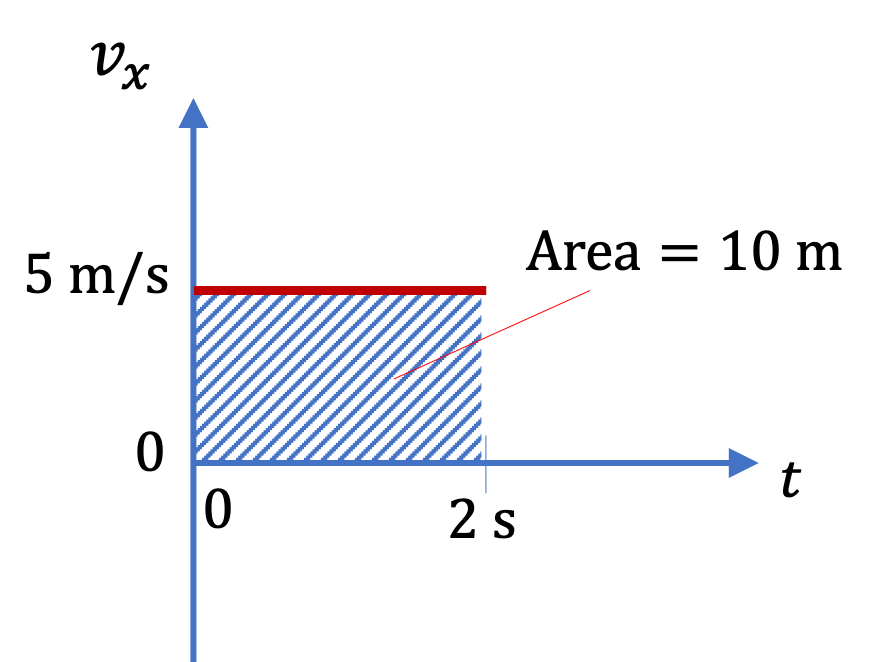If velocity $v_x$ is negative during the interval, it would mean that object is moving towards negative $x$ axis. This shows up as negative area above the plot. Therefore, the $x$ displacement will be $\Delta x = -5\text{ m/s} \times 2\text{ s} = - 10 \text{ m}\text{.}$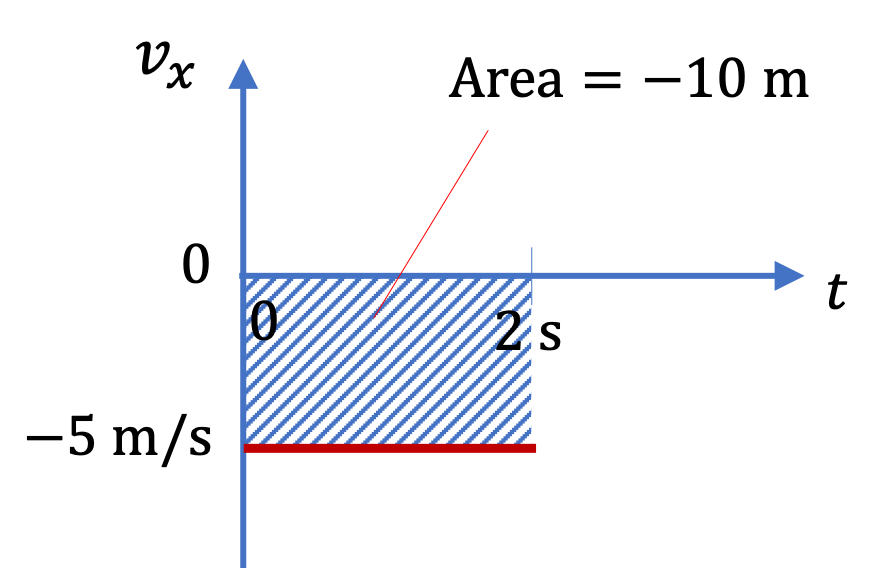In general, we will have three plots, one for $v_x\text{,}$ one for $v_y\text{,}$ and one for $v_z\text{.}$ We then compute $\Delta x\text{,}$ $\Delta y\text{,}$ and $\Delta z\text{,}$ from the net area under the respective plots. Sometimes, we may find that postive areas cancel the negative areas. In that case the displacement will be zero. After we get $\Delta x\text{,}$ $\Delta y\text{,}$ and $\Delta z\text{,}$ we can use displacement vector ad we wish.

\begin{equation*} \Delta \vec r = (\Delta x,\ \Delta y,\ \Delta z ), \text{ or, } \Delta x\, \hat i + \Delta y\, \hat j + \Delta z\, \hat k. \end{equation*}

Numerical illustration of area-under-the-curve and area-above-the-curve used average velocity, whose area is rectangular in shape. For varying velocities, we will encounter areas in the shape of a triangle. The formula for a triangle of height $h$ and base $b$ is

\begin{equation*} A_\triangle = \dfrac{1}{2} b h. \end{equation*}

Figure 4.5.2 shows a $v_x$ versus $t$ plot. Find the displacements in the following intervals, given in seconds, (a) $(0,\ 4)\text{,}$ (b) $(4,\ 6)\text{,}$ (c) $(6,\ 8)\text{,}$ (d) $(8,\ 10)\text{,}$ and (e) $(0,\ 10)\text{.}$

Hint

Compute the areas under the curve, which are just lines here. That makes shapes of the area rectangles and triangles.

(a) $8\text{ m}\text{,}$ (b) $2\text{ m}\text{,}$ (c) $-2\text{ m}\text{,}$ (d) $-4\text{ m}\text{,}$ (e) $4\text{ m}\text{.}$

Solution 1 (a)

(a) Note that the $t$ axis is the $v_x = 0$ line. Therefore, we need the area of the shaded region in the figure on the right. This gives

\begin{align*} \Delta x \amp = 4\text{ s} \times 2\text{ m/s}\\ \amp = 8\text{ m}. \end{align*}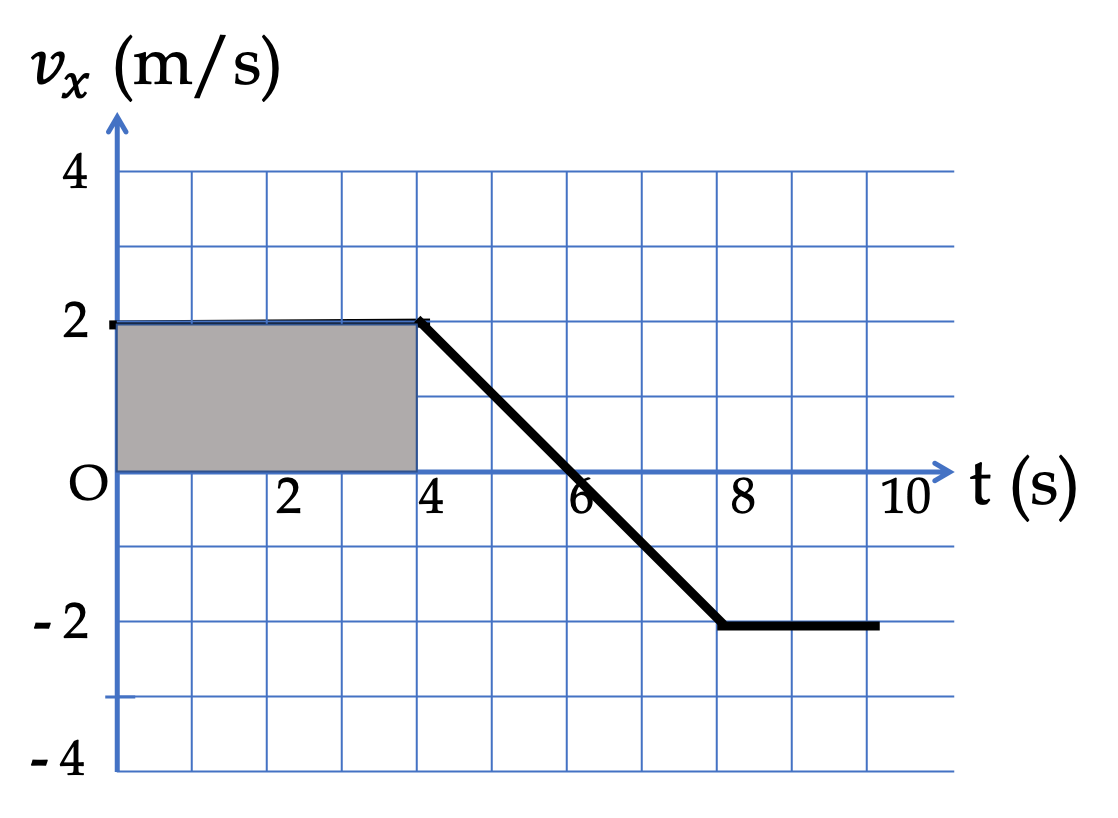Solution 2 (b)

(b) Note that the $t$ axis is the $v_x = 0$ line. Therefore, we need the area of the shaded region in the figure on the right. This gives

\begin{equation*} \Delta x = \dfrac{1}{2}\times 2\text{ s} \times 2\text{ m/s} = 2\text{ m}. \end{equation*}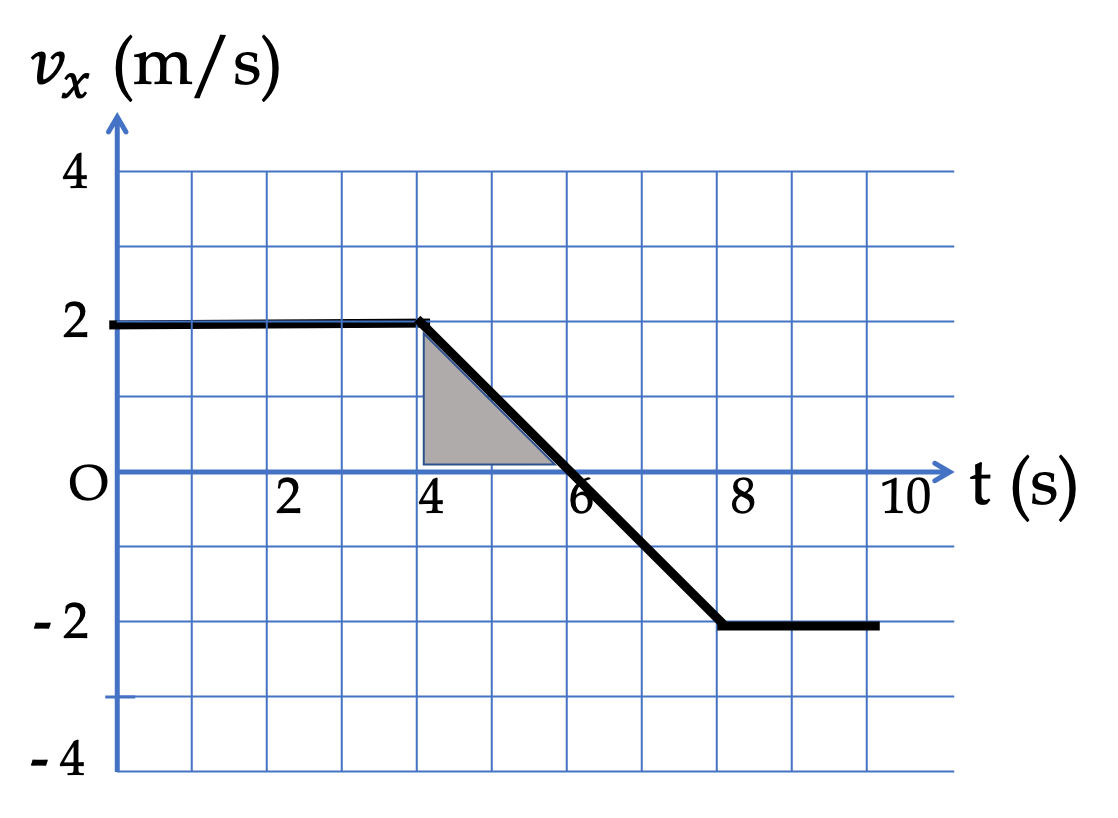Solution 3 (c)

(c) Note that the $t$ axis is the $v_x = 0$ line. Therefore, we need the area of the shaded region in the figure on the right. This area will be negative as is also clear from the direction motion - it is moving to the left. This gives

\begin{align*} \Delta x \amp = \dfrac{1}{2}\times 2\text{ s} \times (-2\text{ m/s})\\ \amp = -2\text{ m}. \end{align*}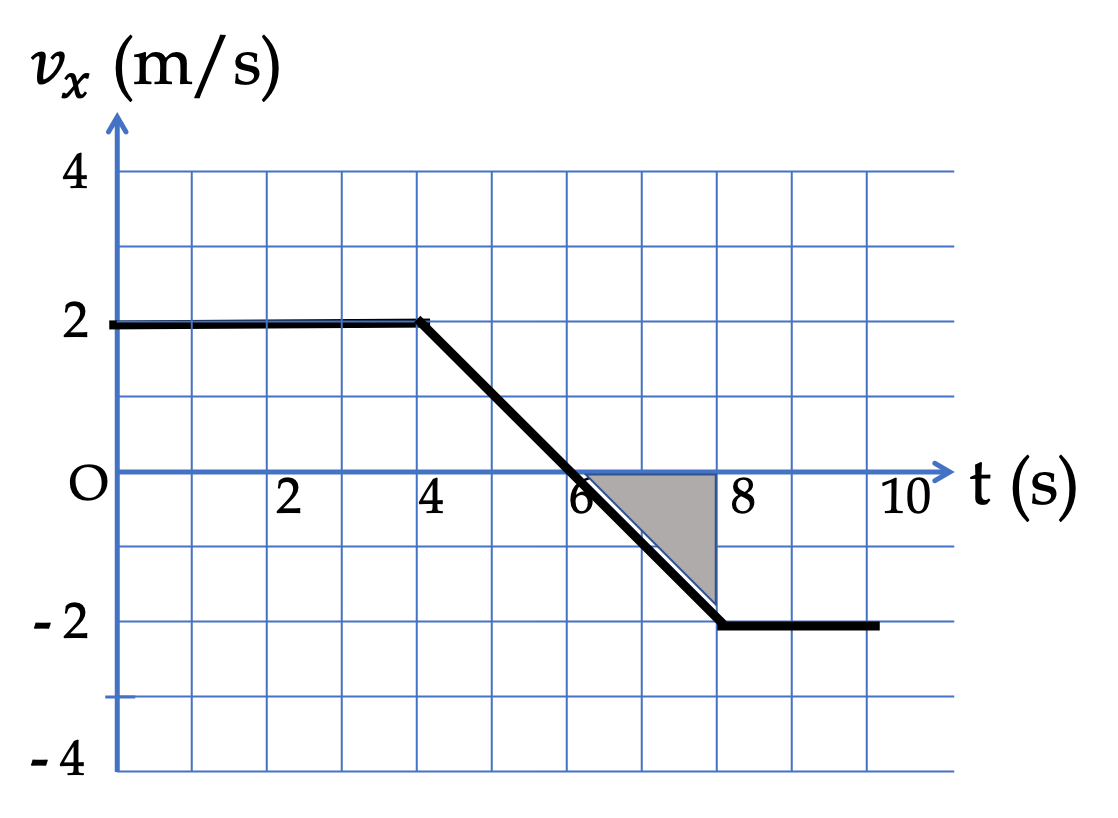Solution 4 (d)

(d) Note that the $t$ axis is the $v_x = 0$ line. Therefore, we need the area of the shaded region in the figure on the right. This area will be negative as is also clear from the direction motion - it is moving to the left. This gives

\begin{align*} \Delta x \amp = 2\text{ s} \times (-2\text{ m/s})\\ \amp = -4\text{ m}. \end{align*}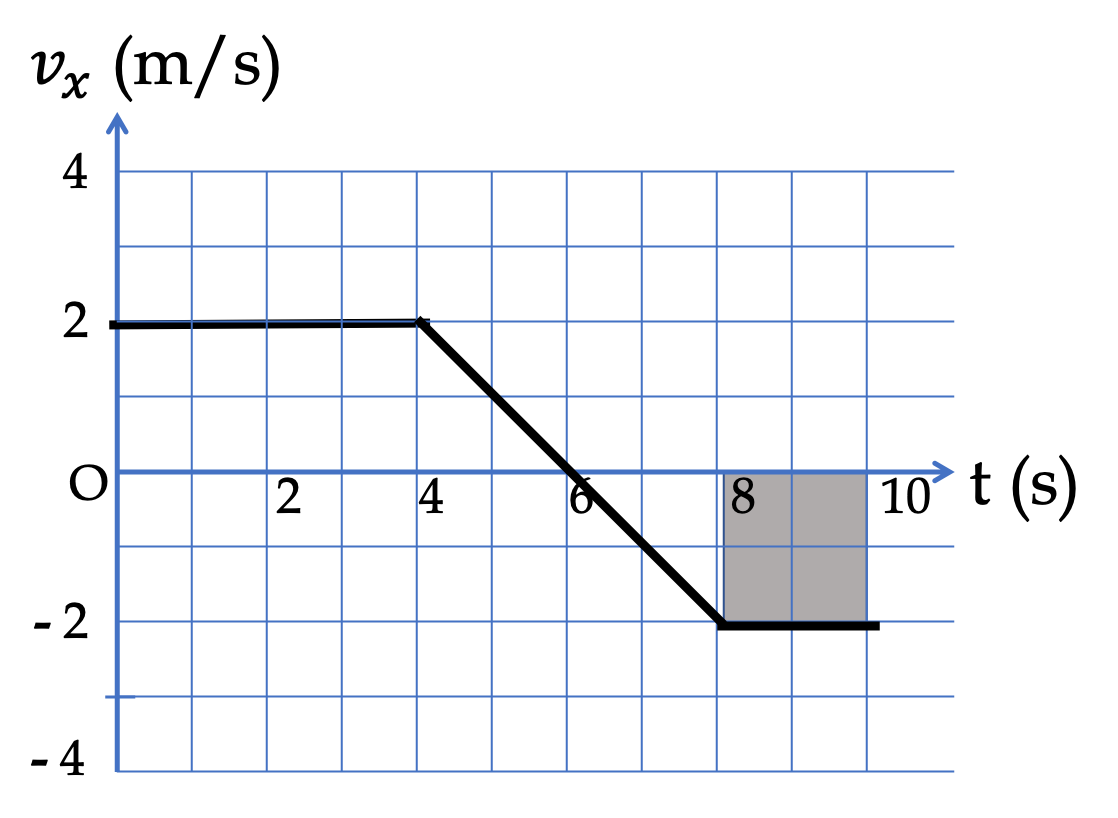Solution 5 (e)

(e) Adding up all the areas we get

\begin{equation*} \Delta x(0\rightarrow 10\text{ s}) = 8\text{ m} + 2\text{ m} + (-2)\text{ m} + (-4)\text{ m} = 4\text{ m}. \end{equation*}

### Subsection4.5.2(Calculus) Integrating the Velocity

Suppose you record the velocity of your car over some period of time. Can you find your final position if you knew where you were at the start of the period?

This question is answerable by using Calculus. In Calculus, there is an inverse relation between derivative and integration.

\begin{equation*} \dfrac{df}{dx} = g \Longleftrightarrow f(x) - f(x_0) = \int_{x_0}^{x}\, g(x)\, dx. \end{equation*}

We can apply this to the $x$ component of the motion to obtain the following for a motion between $t=t_i$ and $t=t_f\text{.}$

\begin{equation} \dfrac{dx}{dt} = v_x \Longleftrightarrow x_f - x_i = \int_{t_i}^{t_f}\, v_x(t)\, dt.\label{eq-displacement-from-velocity-x-fundamental}\tag{4.5.1} \end{equation}

Applying this similarly to $y$ and $z$ motions gives us displacements in those directions as well.

The integral in Eq. (4.5.1) is simple if $v_x$ is constant.

\begin{equation} x_f - x_i = v_x \times (t_f - t_i).\ \ (\text{constant }v_x)\label{eq-displacement-from-velocity-x-constant}\tag{4.5.2} \end{equation}

For non-constant $v_x \text{,}$ we can do the integral analytically if function $v_x(t)$ is simple enough. Otherwise, there are numerical methods for computing the integral. One such numerical method goes by the name, “area under the curve”, which is described below.

A particle is moving in a circle with its velocity components changing with time as given by (in units of m/s with $t$ in units of seconds.)

\begin{equation*} v_x = 5.0\,\cos(2\pi t),\ \ \ v_y = 5.0\,\sin(2\pi t)\text{.} \end{equation*}

(a) What are the components of the displacement vector during the interval from $t=0.25\text{ s}$ to $t=0.50\text{ s} \text{.}$ (b) What are the magnitude and direction of the displacement?

Hint

Integrate velocity components.

(a) $(-0.80\text{ m},\ 0.80\text{ m} )$ (b) $1.13\text{ m}$ in $45^{\circ}$ direction from the negative $x$ axis into the second quadrant.

Solution 1 (a)

(a) The components will be obtained by taking the integral.

\begin{align*} \Delta x \amp = \int_{0.25}^{0.5}\, 5.0\,\cos(2\pi t)\, dt, \\ \amp = \dfrac{5.0}{2\pi}\sin(2\pi t)|_{0.25}^{0.5} \\ \amp = -0.80\text{ m}. \end{align*}
\begin{align*} \Delta y \amp = \int_{0.25}^{0.5}\, 5.0\,\sin(2\pi t)\, dt, \\ \amp = -\dfrac{5.0}{2\pi}\cos(2\pi t)|_{0.25}^{0.5} \\ \amp = 0.80\text{ m}. \end{align*}
Solution 2 (b)

(b) Therefore, the magnitude and angle will be:

\begin{gather*} \Delta r = 0.8\times \sqrt{2} = 1.13\text{ m},\\ \theta = \tan^{-1}(-1) = - 45^{\circ}, \end{gather*}

which we interpret to be clockwise angle of $45^{\circ}$ from the negative $x$ axis into the second quadrant.